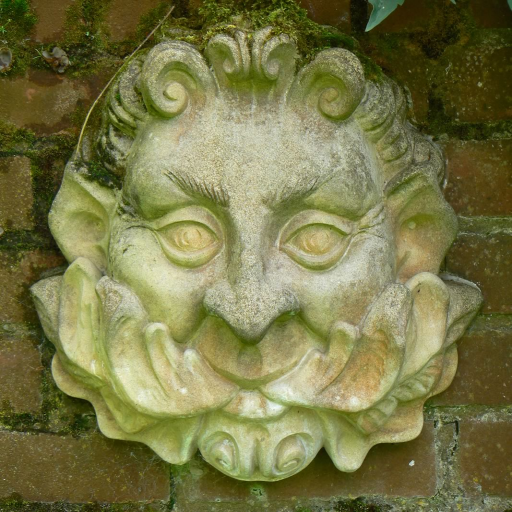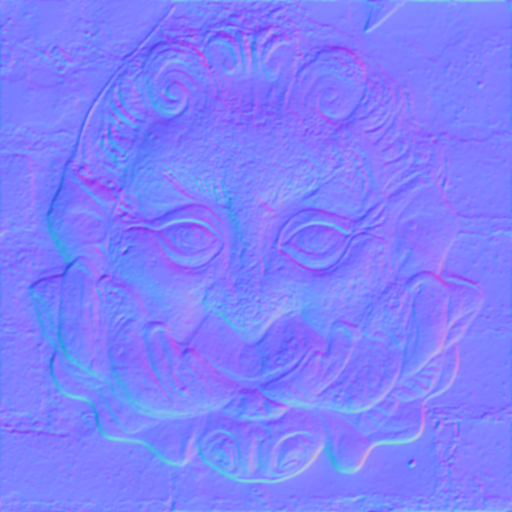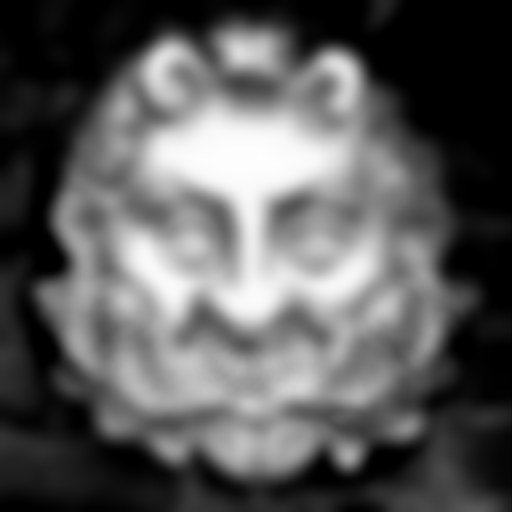###Author Topic: texture mapping?  (Read 4677 times)

####Disastorm

• long
•• Posts: 161##### texture mapping?
« on: July 12, 2011, 11:10:24 pm »
Hello, I don't know much about mapping other than that there are a bunch of mapping styles that make textures look better because of light, but I saw a cool demo on your youtube of parallax mapping on android, so I was wondering what forms of mapping does jpct support and how do you do them (assuming you already generated the mapping images)? The only method I saw related to mapping on Object3D is setBumpMapTexture (and the android one doesn't even have that method?).  How do you do things like height,parallax, normal, displacement, etc?  Are these actually all forms of bumpmapping so it can just be set in that method?  On your youtube I noticed you used an app called ShaderCL to generate the maps.  Does this mean I can use this application to make my textures look better?
« Last Edit: July 12, 2011, 11:15:40 pm by Disastorm »

####EgonOlsen

••• Posts: 12005##### Re: texture mapping?
« Reply #1 on: July 12, 2011, 11:45:57 pm »
Actually, it's not that simple. The setBumpmapTexture()-method is for software renderer only, so this doesn't apply in your case. By default jPCT (as well as -AE) uses the fixed function pipeline, which means that apart from combining textures using multi-texturing with different blending modes, you don't have much more options. That's not saying that you can't create great looking games with that approach though.

However, jPCT, as well as the latest alpha of jPCT-AE, have support for shaders (http://www.jpct.net/wiki/index.php/Shaders). With shaders, you can do almost anything...the drawback is, that you have to do it all in the shader (a little program that looks much like basic C code and that runs on the GPU), which requires a deeper understanding of how the GPU and 3D graphics in general work.

I'll explain in short what the demo on youtube uses for this effect.

At first, we need a normal texture map:For calculating the lighting, we need a normal map:That one can be created out of the texture map by using (for example) ShaderMap CL (CL stands for command line...the tool is free, but has no GUI). What it basically does, is to guess the 3D structure of the texture. That's actually nonsense, but it looks pretty good. Another way it to generate the normal map of a model based on a higher polygon version of that model.

For the additional effect of parallax mapping, i'm using a height map. I made this myself based on a greyscale version of the texture:For the demo, i'm merging the height map and the normal map, so that the height map is stored in alpha channel of the normal map. But that's not needed, it just saves texture space.

Then you need a shader to make something of this. The shader consists of two parts, a vertex shader and a fragment shader.

Code: [Select]
`uniform mat4 modelViewMatrix;uniform mat4 modelViewProjectionMatrix;uniform vec4 additionalColor;uniform vec4 ambientColor;uniform vec3 lightPositions;attribute vec4 position;attribute vec3 normal;attribute vec4 tangent;attribute vec2 texture0;varying vec3 lightVec; varying vec3 eyeVec;varying vec2 texCoord; void main(void){ texCoord = texture0.xy; vec3 n = normalize(modelViewMatrix * vec4(normal,0.0)).xyz; vec3 t = normalize(modelViewMatrix * vec4(tangent.xyz, 0.0)).xyz; vec3 b = tangent.w*cross(n, t); vec3 vVertex = vec3(modelViewMatrix * position); vec3 tmpVec = lightPositions.xyz - vVertex; vec3 lv; vec3 ev; lv.x = dot(tmpVec, t); lv.y = dot(tmpVec, b); lv.z = dot(tmpVec, n); lightVec=lv; tmpVec = vVertex*-1.0; eyeVec.x = dot(tmpVec, t); eyeVec.y = dot(tmpVec, b); eyeVec.z = dot(tmpVec, n); gl_Position = modelViewProjectionMatrix * position;}`
...and this is the fragment shader:
Code: [Select]
`precision mediump float;varying vec3 lightVec;varying vec3 eyeVec;varying vec2 texCoord;uniform sampler2D textureUnit0;uniform sampler2D textureUnit1;uniform vec3 diffuseColors;uniform vec3 specularColors;uniform vec4 ambientColor;uniform float invRadius;uniform float heightScale;void main (){ vec4 vAmbient = ambientColor; vec3 vVec = normalize(eyeVec); float height = texture2D(textureUnit1, texCoord).a; vec2 offset = vVec.xy * (height * 2.0 - 1.0) *heightScale; vec2 newTexCoord = texCoord + offset; vec4 base = texture2D(textureUnit0, newTexCoord); vec3 bump = normalize(texture2D(textureUnit1, newTexCoord).xyz * 2.0 - 1.0); float distSqr = dot(lightVec, lightVec); float att = clamp(1.0 - invRadius * sqrt(distSqr), 0.0, 1.0); vec3 lVec = lightVec * inversesqrt(distSqr); float diffuse = max(dot(lVec, bump), 0.0); vec4 vDiffuse = vec4(diffuseColors,0) * diffuse; float specular = pow(clamp(dot(reflect(-lVec, bump), vVec), 0.0, 1.0), 0.85); vec4 vSpecular = vec4(specularColors,0) * specular; gl_FragColor = (vAmbient*base + vDiffuse*base + vSpecular) * att*2.0;}`
That's all...but as you can see, it's more work than just using the fixed function pipeline with it's limited but easier to use capabilities. Also note that this example is for jPCT-AE, i.e. for OpenGL ES 2.0. For jPCT, the shader would look a little different because it hooks into the fixed function pipeline and has access to some attributes of it while the AE-version has to set them itself. Also, desktop jPCT currently has no option to compute the tangent vectors that you need for this shader while AE has. The shader example in the wiki works around this by computing them in the shader, but that's only an approximation which works good enough for simple bump mapping shaders but not for parallax mapping.

As an example of a shader for desktop jPCT, i'll post the phong lighting shader that i'm using in Robombs for the players.

Code: [Select]
`varying vec3 normal, lightDir, eyeVec;varying vec2 texCoord; void main(){ normal = gl_NormalMatrix * gl_Normal; vec3 vVertex = vec3(gl_ModelViewMatrix * gl_Vertex); lightDir = vec3(gl_LightSource.position.xyz - vVertex); eyeVec = -vVertex; gl_Position = ftransform(); texCoord = gl_MultiTexCoord0.xy;}`
Code: [Select]
`varying vec3 normal, lightDir, eyeVec;varying vec2 texCoord;uniform sampler2D colorMap; const float cos_outer_cone_angle = 0.8; // 36 degreesvoid main (void){ vec4 base = texture2D(colorMap, texCoord); vec4 final_color = (gl_FrontLightModelProduct.sceneColor * gl_FrontMaterial.ambient) + (gl_LightSource.ambient * gl_FrontMaterial.ambient); vec3 L = normalize(lightDir); vec3 D = normalize(gl_LightSource.spotDirection); float cos_cur_angle = dot(-L, D); float cos_inner_cone_angle = gl_LightSource.spotCosCutoff; float cos_inner_minus_outer_angle = cos_inner_cone_angle - cos_outer_cone_angle; if (cos_cur_angle > cos_inner_cone_angle) { vec3 N = normalize(normal); float lambertTerm = max( dot(N,L), 0.0); if(lambertTerm > 0.0) { final_color += gl_LightSource.diffuse * gl_FrontMaterial.diffuse * lambertTerm; vec3 E = normalize(eyeVec); vec3 R = reflect(-L, N); float specular = clamp(pow( max(dot(R, E), 0.0), gl_FrontMaterial.shininess ),0.001,1.0); final_color += gl_LightSource.specular * gl_FrontMaterial.specular * specular; } } else if( cos_cur_angle > cos_outer_cone_angle ) { float falloff = (cos_cur_angle - cos_outer_cone_angle) / cos_inner_minus_outer_angle; vec3 N = normalize(normal); float lambertTerm = max( dot(N,L), 0.0); if(lambertTerm > 0.0) { final_color += gl_LightSource.diffuse * gl_FrontMaterial.diffuse * lambertTerm * falloff; vec3 E = normalize(eyeVec); vec3 R = reflect(-L, N); float specular = clamp(pow( max(dot(R, E), 0.0), gl_FrontMaterial.shininess ),0.001,1.0); final_color += gl_LightSource.specular * gl_FrontMaterial.specular * specular * falloff; } } gl_FragColor = final_color*2*base; gl_FragColor.a=0.45;}`
Final words: I recommend to go with the fixed function pipeline first. Results can look great with proper artwork. Shaders introduce a whole new domain that isn't particularly easy to understand IMHO.

####Disastorm

• long
•• Posts: 161##### Re: texture mapping?
« Reply #2 on: July 13, 2011, 03:27:08 am »
Thanks for the long response.  The shaders stuff sounds pretty complicated as I've never read into them before.  I had just thought I could generate the maps with ShaderCL and apply them but I guess thats not the case.  Maybe some time in the future though I'll try to read about the Shader thing since it seems like they make things look alot better.  I will also read about multitexturing since I didn't know about that.
« Last Edit: July 13, 2011, 03:36:49 am by Disastorm »